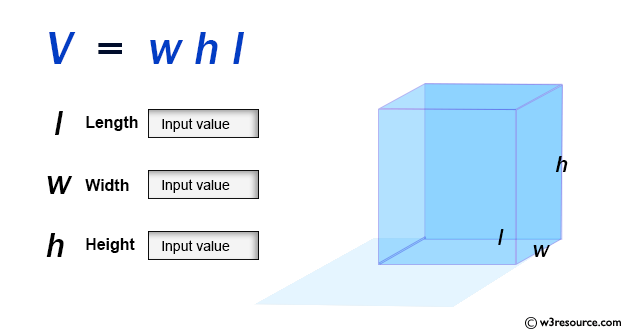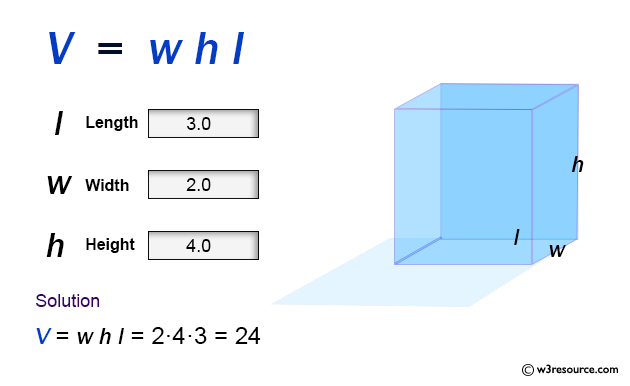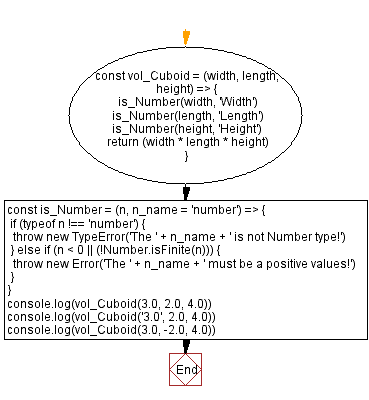# JavaScript: Volume of a Cuboid

## JavaScript Math: Exercise-56 with Solution

Write a JavaScript program to calculate the volume of a Cuboid.

From Wikipedia –
In geometry, a cuboid is a hexahedron, a six-faced solid. Its faces are quadrilaterals. Cuboid means "like a cube", in the sense that by adjusting the length of the edges or the angles between edges and faces a cuboid can be transformed into a cube.

Sample Data:Sample Solution:

HTML Code:

``````<!DOCTYPE html>
<html>
<meta charset="utf-8">
<title>JavaScript function to Volume of a Cuboid</title>
<body>

</body>
</html>
```
```

JavaScript Code:

``````const vol_Cuboid = (width, length, height) => {
is_Number(width, 'Width')
is_Number(length, 'Length')
is_Number(height, 'Height')
return (width * length * height)
}
const is_Number = (n, n_name = 'number') => {
if (typeof n !== 'number') {
throw new TypeError('The ' + n_name + ' is not Number type!')
} else if (n < 0 || (!Number.isFinite(n))) {
throw new Error('The ' + n_name + ' must be a positive values!')
}
}
console.log(vol_Cuboid(3.0, 2.0, 4.0))
console.log(vol_Cuboid('3.0', 2.0, 4.0))
console.log(vol_Cuboid(3.0, -2.0, 4.0))
```
```

Sample Output:

```24
-------------------------------------------------------
Uncaught TypeError: The Width is not Number type!
at https://cdpn.io/cpe/boomboom/pen.js?key=pen.js-806436e9-e230-3eac-27f1-87bb5a3ce22e:9
--------------------------------------------------------------------------------------------
Uncaught Error: The Length must be a positive values!
at https://cdpn.io/cpe/boomboom/pen.js?key=pen.js-af547994-8231-4e1e-2596-c048d68af34f:11
```

Flowchart:Live Demo:

See the Pen javascript-math-exercise-56 by w3resource (@w3resource) on CodePen.

Improve this sample solution and post your code through Disqus

Previous: Swap two variables.
Next: Volume of a Cube.

What is the difficulty level of this exercise?

Test your Programming skills with w3resource's quiz.

﻿